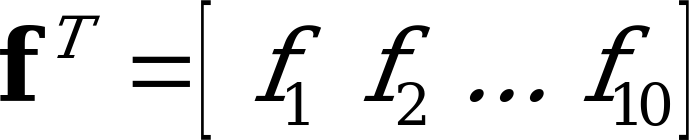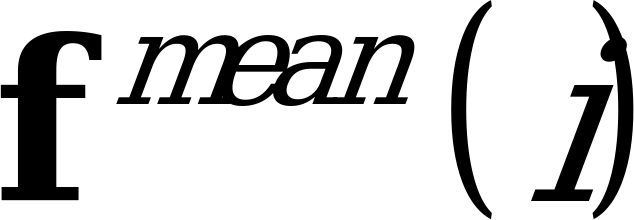5 Functions on the transmit (TX) side

06.923GPPComfort noise aspects for Adaptive Multi-Rate speech traffic channelsTS

The comfort noise evaluation algorithm uses the following parameters of the AMR speech encoder, defined in GSM 06.90 :

– the unquantized Linear Prediction (LP) parameters, using the Line Spectral Pair (LSP) representation, where the unquantized Line Spectral Frequency (LSF) vector is given by;

– the unquantized LSF vector for the 12.2 kbit/s mode is given by the second set of LSF parameters in the frame.

The algorithm computes the following parameters to assist in comfort noise generation:

– the averaged LSF parameter vector(average of the LSF parameters of the eight most recent frames);

– the averaged logarithmic frame energy (average of the logarithmic energy of the eight most recent frames).

These parameters give information on the level () and the spectrum () of the background noise.

The evaluated comfort noise parameters (and) are encoded into a special frame, called a Silence Descriptor (SID) frame for transmission to the RX side.

A hangover logic is used to enhance the quality of the silence descriptor frames. A hangover of 7 frames is added to the VAD flag so that the coder waits with the switch from active to inactive mode for a period of 7 frames, during that time the decoder can compute a silence descriptor frame from the quantized LSFs and the logarithmic frame energy of the decoded speech signal. Therefore, no comfort noise description is transmitted in the first SID frame after active speech. If the background noise contains transients which will cause the coder to switch to active mode and then back to inactive mode in a very short timeperiod, no hangover is used. Instead the previously used comfort noise frames are used for comfort noise generation.

The first SID frame also serves to initiate the comfort noise generation on the receive side, as a first SID frame is always sent at the end of a speech burst, i.e., before the radio transmission is terminated.

The scheduling of SID or speech frames on the radio path is described in GSM 06.93 .

5.1 LSF evaluation

The comfort noise parameters to be encoded into a SID frame are calculated over consecutive frames marked with VAD=0, as follows:

The averaged LSF parameter vector of the frame i shall be computed according to the equation:

(1)

where is the (unquantized) LSF parameter vector of the current frame i () and past frames ().

The averaged LSF parameter vectorof the frame i is encoded using the same encoding tables that are also used by the 7.4 kbit/s mode for the encoding of the non-averaged LSF parameter vectors in ordinary speech encoding mode, but the quantization algorithm is modified in order to support the quantization of comfort noise.

The LSF parameter prediction residual to be quantized for frame i is obtained according to the following equation:

(2)

where is a reference vector picked from a codebook.

The vector used in eq (2) is encoded for each SID frame. A lookup table containing 8 vectors typical for background noise are searched. The vector which yields the lowest prediction residual energy is selected. After the above step the LSF parameter encoding procedure is performed. The 3-bit index for the reference vector and the 26 bits for LSF parameter are transmitted in the SID frame (see bit allocation in table 1).

5.2 Frame energy calculation

The frame energy is computed for each frame marked with VAD=0 according to the equation :

(3)

where is the HP-filtered input speech signal of the current frame i.

The averaged logarithmic energy is computed by:

. (4)

The averaged logarithmic energy is quantized means of a 6 bit algorithmic quantizer. The 6 bits for the energy index are transmitted in the SID frame (see bit allocation in table 1).

5.3 Modification of the speech encoding algorithm during SID frame generation

When the TX_TYPE is not equal to SPEECH the speech encoding algorithm is modified in the following way:

– the non-averaged LP parameters which are used to derive the filter coefficients of the filtersandof the speech encoder are not quantized;

– the open loop pitch lag search is performed, but the closed loop pitch lag search is inactivated. The adaptive codebook gain and memory is set to zero;

– no fixed codebook search is made;

– the memory of weighting filteris set to zero, i.e., the memory ofis not updated;

– the ordinary LP parameter quantization algorithm is inactive. The averaged LSF parameter vectoris calculated each time a new SID frame is to be sent to the Radio Subsystem. This parameter vector is encoded into the SID frame as defined in subclause 5.1;

– the ordinary gain quantization algorithm is inactive;

– the predictor memories of the ordinary LP parameter quantization and fixed codebook gain quantization algorithms are initialized when TX_TYPE is not SPEECH, so that the quantizers start from known initial states when the speech activity begins again.

5.4 SID-frame encoding

The encoding of the 35 comfort noise bits in a SID frame is described in GSM 05.03 where the encoding of the first SID frame is also described. The bit allocation and sequence of the bits from comfort noise encoding is shown in table 1.